# Zoom Scatter Chart

This chart type belongs to FusionCharts XT.

The FusionCharts Suite XT zoom-scatter chart, a special type of chart, is an extension of the scatter chart with the zooming and panning features and the ability to display millions of data points because of these features.

The zoom-scatter chart is primarily used to find correlations between datasets. Correlations in the chart can be determined by visual patterns in the data. Higher the number of data points, higher is the accuracy of the correlations. The zoom-scatter chart is capable of handling more than a million data points in modern canvas supported browsers (including IE9+).

## Create a Zoom Scatter Chart

Let's create a chart which compares the admission rate (in percent) with the average annual returns on the degree (in percent) for five majors and over a period of 20 years.

To create a zoom scatter chart follow the steps given below:

• In the JSON data, set the attributes and their corresponding values in `"<attributeName>": "<value>"` format.

• Specify the chart type using the `type` attribute. To render a zoom scatter chart, set `zoomscatter`.

• Set the container object using `renderAt` attribute.

• Specify the dimension of the chart using `width` and `height` attributes.

• Set the type of data (JSON/XML) you want to pass to the chart object using `dataFormat` attribute.

Some of the attributes used to create a zoom scatter chart are:

• `id` attribute sets the unique id for the data plot.

• `x` attribute specifies the x-coordinate of the data plot.

• `y` attribute specifies the y-coordinate of the data plot.

The above attributes belong to the `data` object (child of the dataset object).

For a detailed list of attributes, refer to the chart attributes page of zoom scatter chart.

Take a look at the sample zoom-scatter chart shown below:

FusionCharts will load here..

Click here to edit the zoom-scatter chart.

Drag the cursor over the chart to select and zoom into a subset of data points.

At the time of rendering, the zoom-scatter chart is displayed with a macroscopic view of data. To select a subset of data points and zoom into them, drag the mouse pointer over the data points. A rectangle is drawn to highlight the selected data points and all points within this rectangle are zoomed.

When a subset of data plots is selected and zoomed into, the selected portion expands to occupy the entire width and height of the chart. Data is neatly compressed so that all of it fits into the height and width of the chart. To analyze the data in greater detail, the select and zoom process can be repeated several times over, until the last level of granularity. The chart can be restored to its original macroscopic display mode by clicking the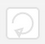button on the toolbar.

For a large amount of data rendered within limited chart space, this chart helps you identify the correlation between data as well as analyze it further by zooming and panning into the data.

## The Zoom-scatter Chart Toolbar

The zoom-scatter chart toolbar is an important interaction mode for the users of this chart. The toolbar, shown in the image below, is located in the top-right corner of the chart.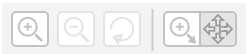The table below briefly describes the buttons on the toolbar of a zoom-scatter chart.

Button Icon Button Name Function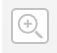Zoom-in Click to zoom-in to the chart/selected subset of data by a predefined amount, based on the center pivot point of the chart/dataset.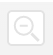Zoom-out Click to zoom-out of the chart/selected subset of data by a predefined amount, similar to the zoom-in functionality.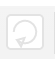Reset Click to zoom-out to the original macroscopic view of data.Select-zoom/Pan switch button Click to switch between the modes of interaction. The zoom-scatter chart supports two modes of interaction—the zoom mode and the pan mode. The zoom mode lets you select a subset of data to get a zoomed view of that data. The pan mode lets you pan through the data in the current chart view. By default, the zoom mode is enabled. After every zoom, the pan mode is activated.

In order to render zoom scatter chart, include fusioncharts.zoomscatter.js in the project folder with rest of the .js files as mentioned here.

The anchors in the zoom-scatter chart cannot be made clickable because FusionCharts does not include the supporting API for defining an external URL for the data points due to performance concerns.

Now, let's see how to draw regression lines in the zoom scatter chart.

## Draw Regression Lines

A regression line, rendered as a straight line, is used to show the trend of y values for the x values or the trend of x values for the y values. A regression line, therefore, can be used to derive a particular trend from the scattered data points in the chart canvas and predict values accordingly. For example, regression lines can be used to find trends and predict future sales, stock prices, currency exchange rates, productivity gains resulting from a training program, and so on.

There are several methods to calculate and draw regression lines; the zoom-scatter chart, using linear regression, use the least-squares deviation method (also known as the least absolute deviation method). This method calculates the best-fitting straight-line for the observed data by minimizing the sum of the squares of the vertical deviation of each data point from the line (if a point lies on the fitted line exactly, then its vertical deviation is 0). As the deviation values are first squared and then summed, there are no cancellations between positive and negative values.

To draw the regression line in a zoom scatter chart, set the `showRegressionLine` attribute to `1`. The default value for this attribute is `0`.

Refer to the code given below:

``````{
"chart": {
...
},
"dataset": [{
"showRegressionLine" : "1"
}]
}``````

A zoom-scatter chart rendered with regression lines is shown below:

FusionCharts will load here..

Click here to edit the zoom scatter chart.

### Modes of Regression

Regression lines can be drawn in one of the following two modes:

• Y on X: (Default mode) Used when y values are predicted, or a trend of y values is calculated, based on the xvalues

• X on Y: Used when x values are predicted, or a trend of x values is calculated, based on the y values

In the zoom-scatter chart shown above, the regression lines are drawn in the Y on X mode.

Here, let's try out the same chart with the regression lines drawn using the X on Y mode. A brief description of the attributes used to set the mode and customize regression lines are:

• Set the `showYonX` attribute to `0` to draw the regression lines in X on Y mode. The default value for this attribute is `1`, which draws the regression lines in Y on X mode. This attribute belongs to the `dataset` object.

• To set the color in which regression line will be drawn, specify the hex color code to `regressionLineColor` attribute.

• Set the thickness of the regression line using `regressionLineThickness` attribute.

• Set the transparency of the regression line using `regressionLineAlpha` attribute.

The chart will look like as shown below:

FusionCharts will load here..